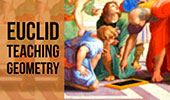# Online Geometry: Triangle Centers, Theorems and Problems Index, Page 4

 Triangle Centers - Table of Content 4Proposed Problem 79: Triangle. Similarity, Altitudes, Orthocenter, Incircles, Inradii. Interactive Orthopole of a Triangle: Using TracenPoche Dynamic Geometry Software, Online Step-by-Step construction, manipulation, and animation. Morley's Triangle & Center: with interactive animation and manipulation. Schiffler Point: Four Euler Lines with interactive animation and manipulation. Incenter, Excenter, Incircle, Excircle Using TracenPoche Dynamic Software Step-by-Step construction, Manipulation, and animation. Three Tangent Circles Theorem Using TracenPoche Dynamic Software Step-by-Step construction, Manipulation, and animation. Sangaku Geometry Theorem: Cyclic Quadrilateral. Inradii, Rectangle. Sangaku Problem (An Old Japanese Theorem). Inradii, Carnot's theorem. Triangle Centers. Word find puzzle. Feuerbach Theorem One of the most striking theorems in triangle geometry. Feuerbach Points and Nine-Point Circle with interactive animation, manipulation, and step-by-step construction. Proposed Problem 51: Fagnano's Problem Inscribed Triangle with the Minimum Perimeter. Proposed Problem 40. Triangle, Incenter, Excenter, Angles 80, 40, Distances. Proposed Problem 39. Triangle, Incircle, Bisector, Cyclic Quadrilateral and angles. Proposed Problem 39 Geometry Help. Facts you should know for the proposed problem 39. Proposed Problem 38. Right Triangle, altitude, incircles, incenters, and angles. Proposed Problem 37. Right Triangle, altitude, incircles, incenters, and orthocenter. Proposed Problem 36. Right Triangle, altitude, incircles and inradii. Proposed Problem 34. Right triangle, cevian, incircles, tangents and inradius.

Go to Page: Previous | 1 | 2 | 3 | 4 | 5 | Next

 Home | Sitemap | Search | Geometry | Email | by Antonio Gutierrez